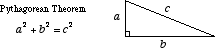# pythagorean theorem

Pythagorean Theorem

An equation relating the lengths of the sides of a right triangle. The sum of the squares of the legs of a right triangle is equal to the square of the hypotenuse.